# NCERT Exemplar Class 10 Science Solutions for Chapter 13 - Magnetic Effects Of Electric Current

## NCERT Exemplar Solutions Class 10 Science Chapter 13 – Free PDF Download

NCERT Exemplar Solutions for Class 10 Science Chapter 13 Magnetic Effects of Electric Current are an essential study tool for the students to pass the CBSE Class 10 examination with flying colours. This study material presents you with the answers to the questions provided in the NCERT Exemplar book. NCERT Exemplar aids you in gaining precise knowledge on different kinds of questions to be asked in the examinations.

Chapter 13 in the Class 10 Science Textbook deals with the study of magnetic fields and electromagnetic effects. In this chapter, students will study about electromagnets, electric motors, electric generators and the magnetic effect of electric current as well as moving magnets. However, the main objective of the chapter is to help students gain practical knowledge about electrical current and its magnetic effects.

### Download the PDF of NCERT Exemplar for Class 10 Science Chapter 13 – Magnetic Effects of Electric Current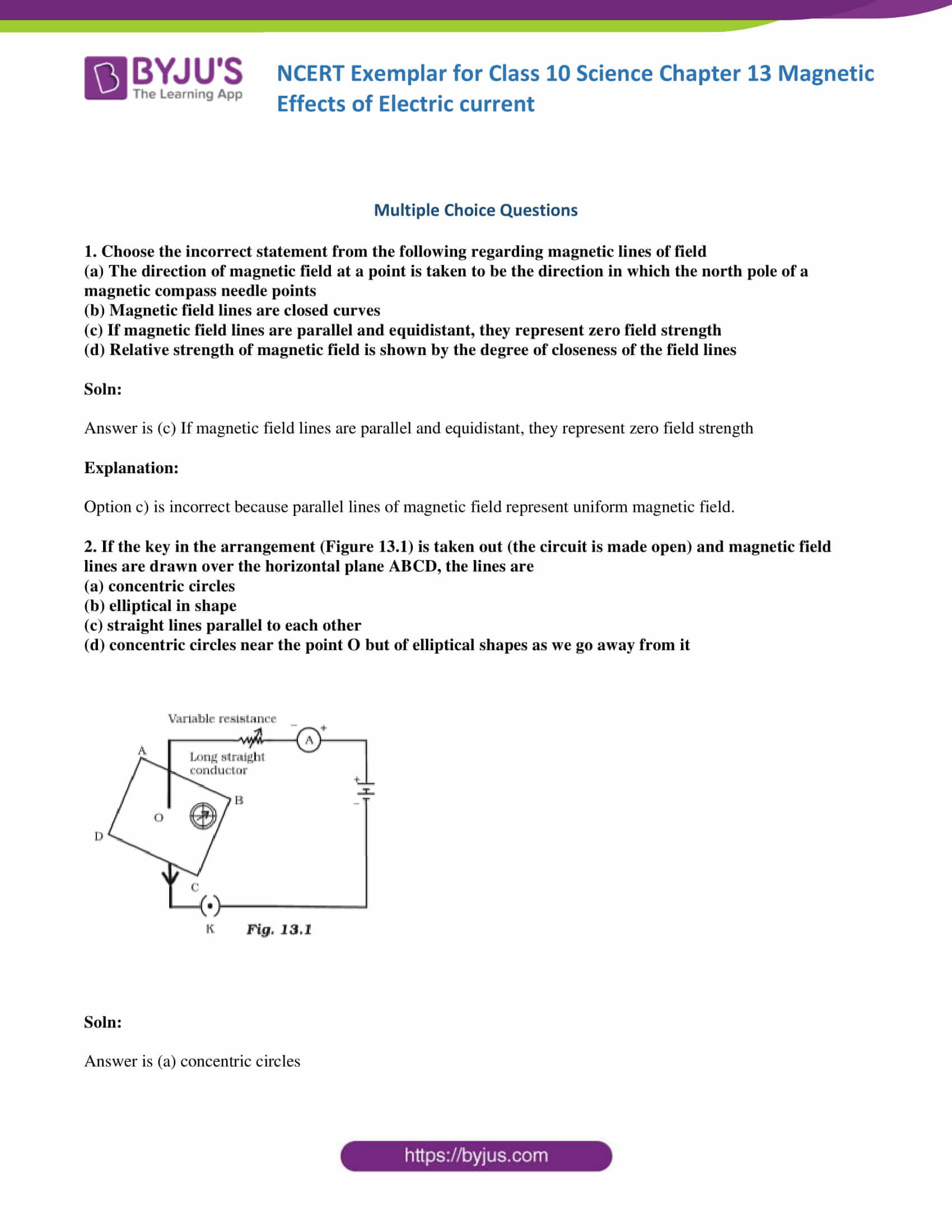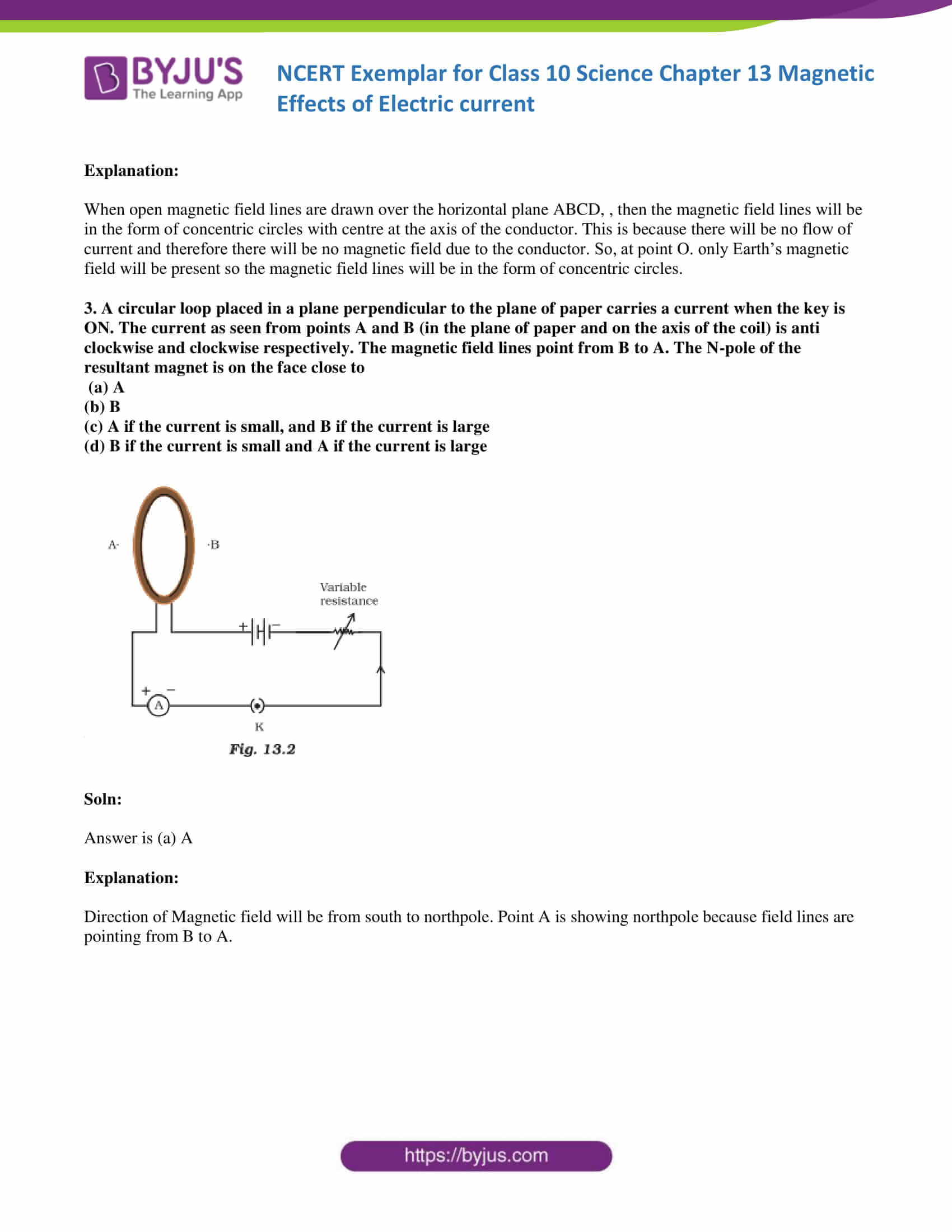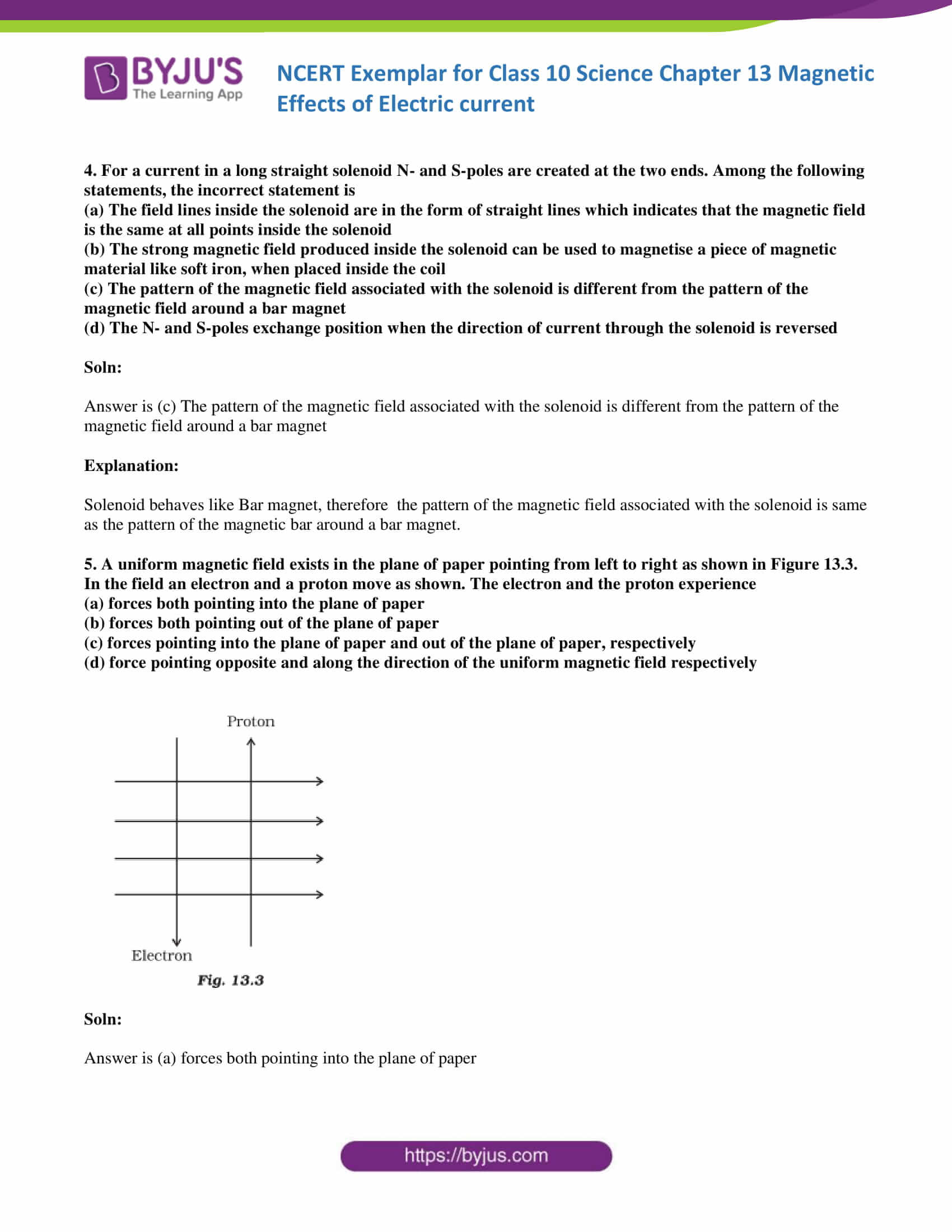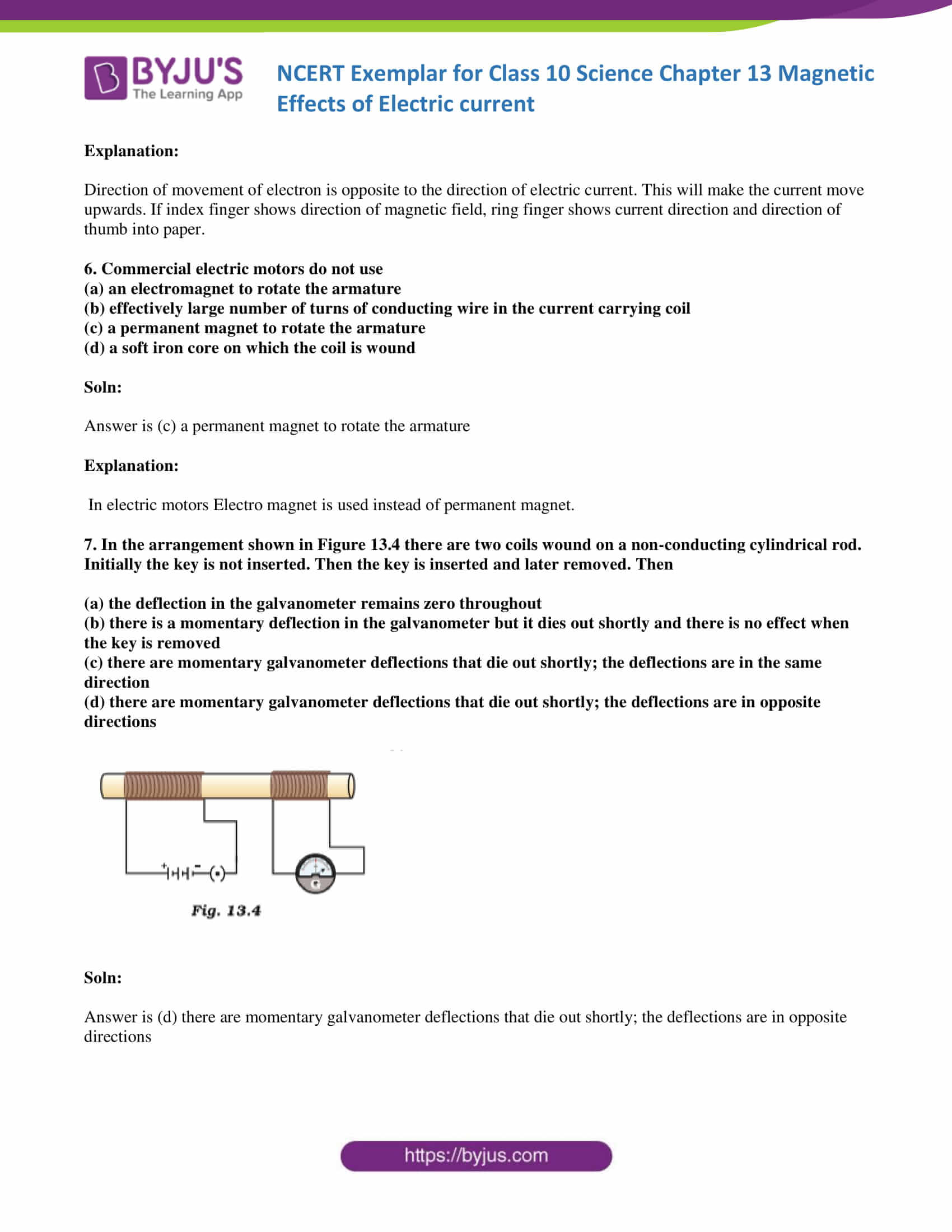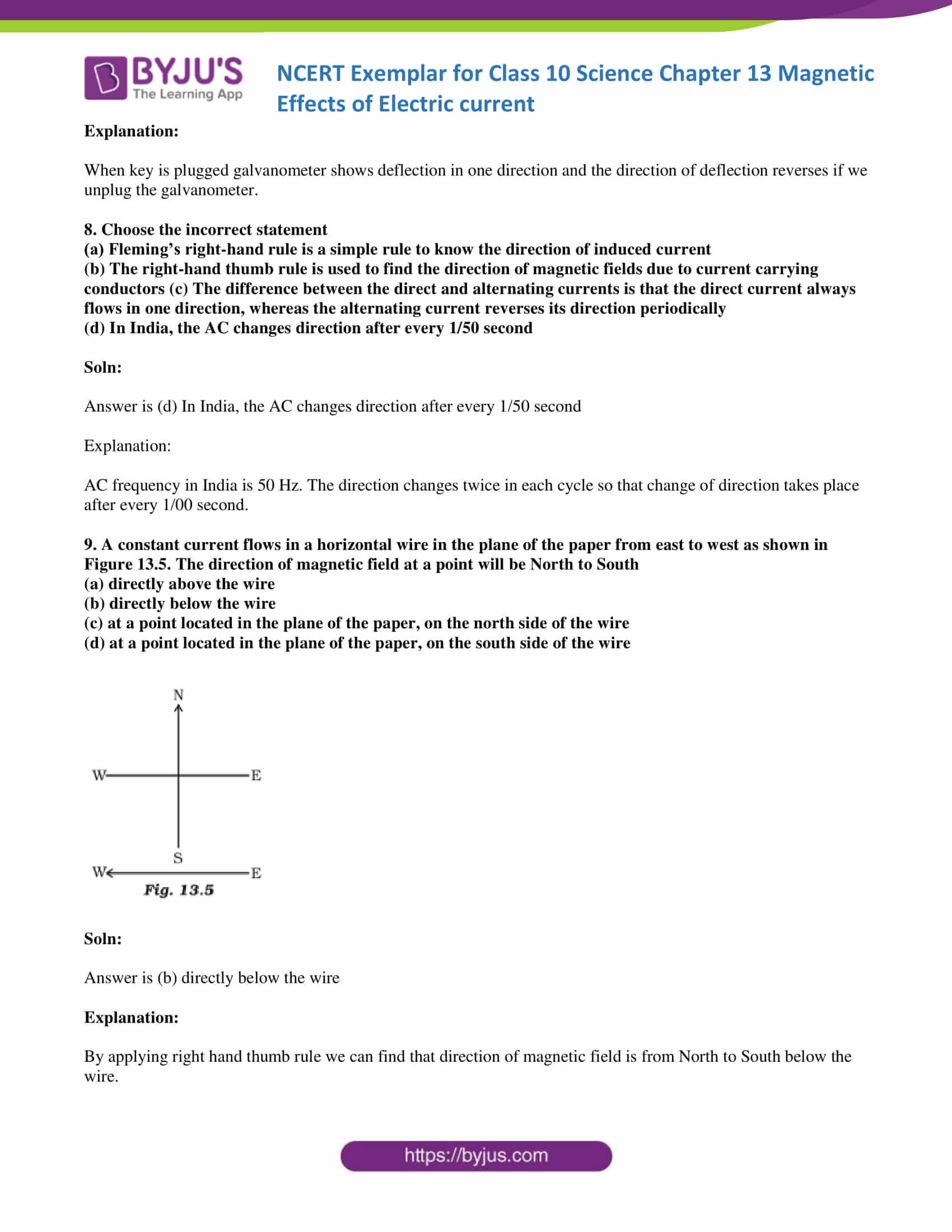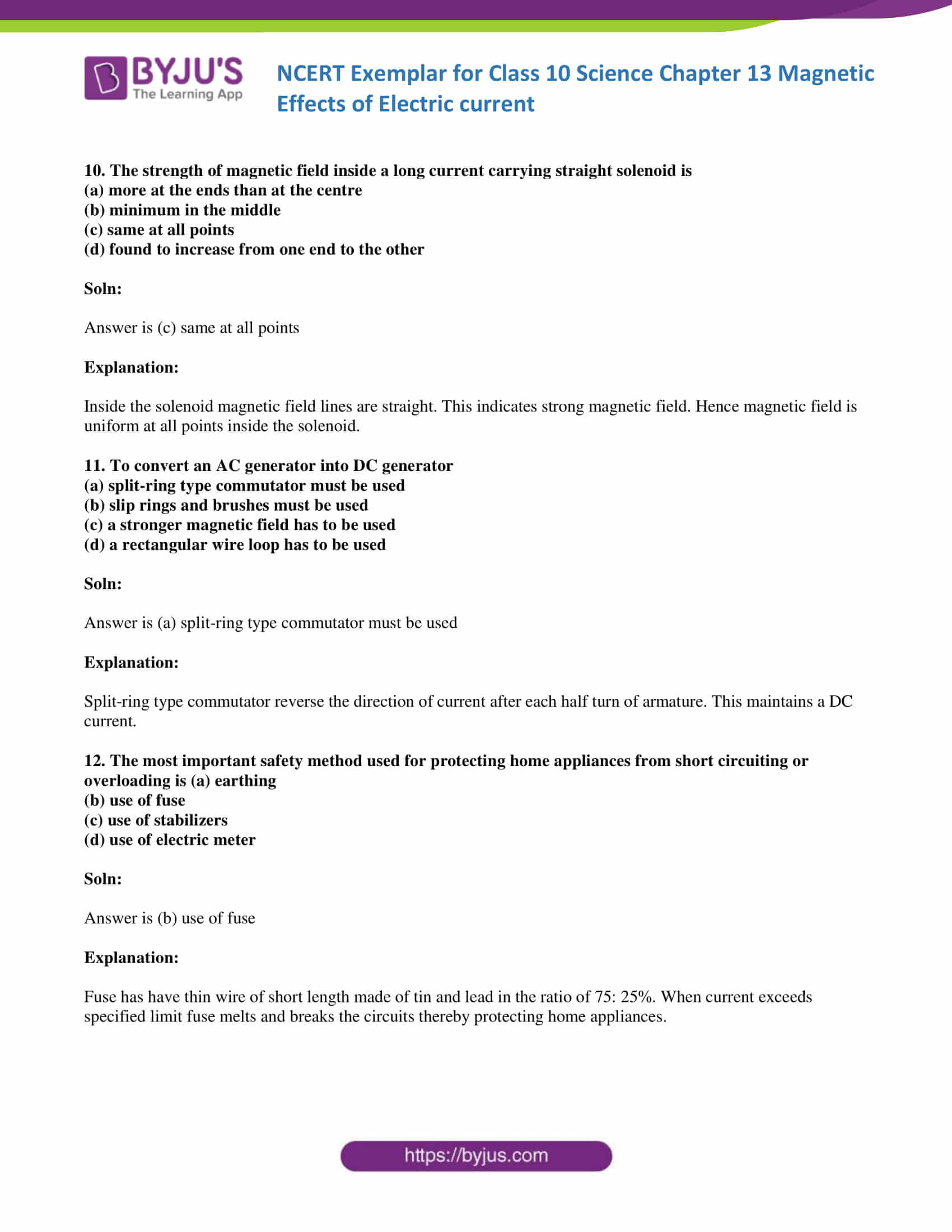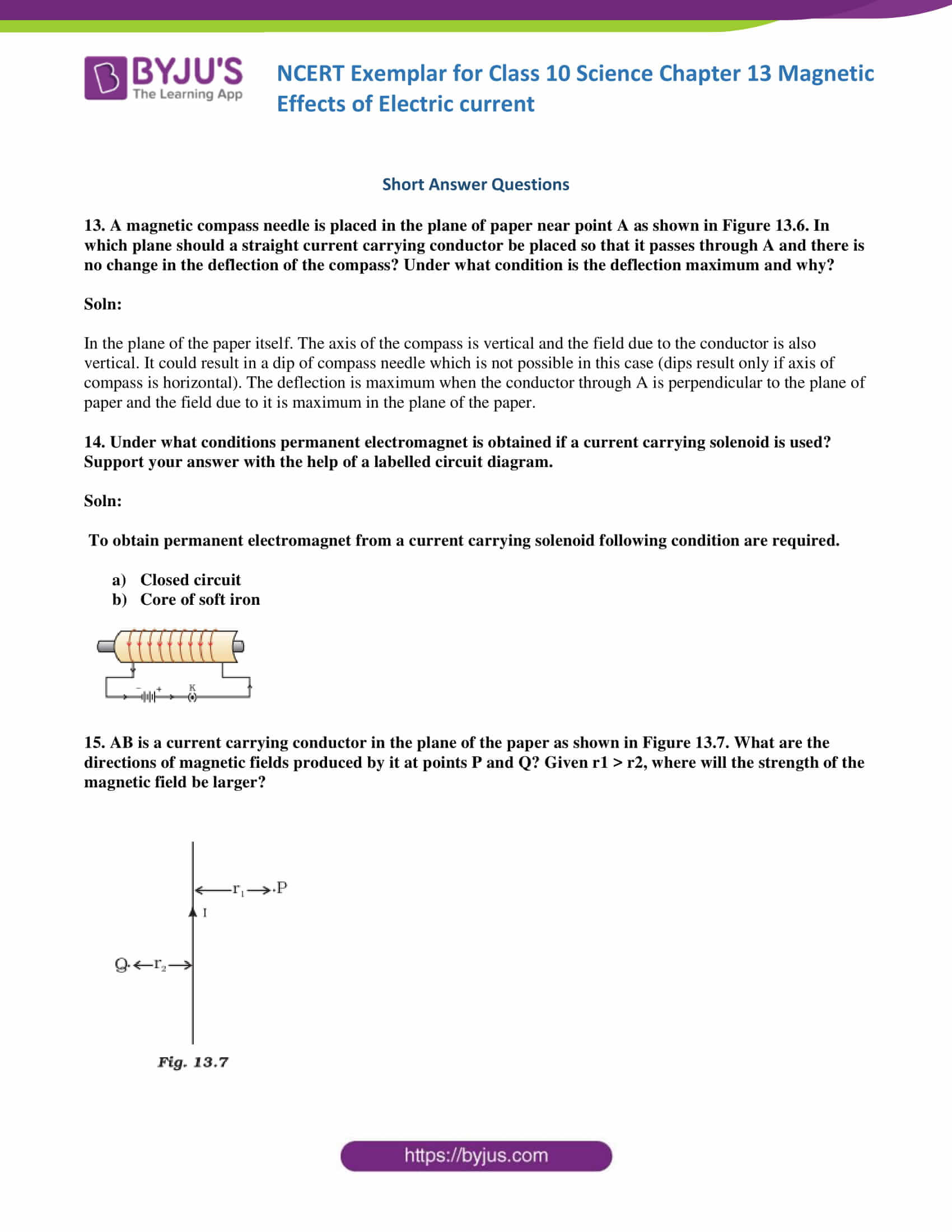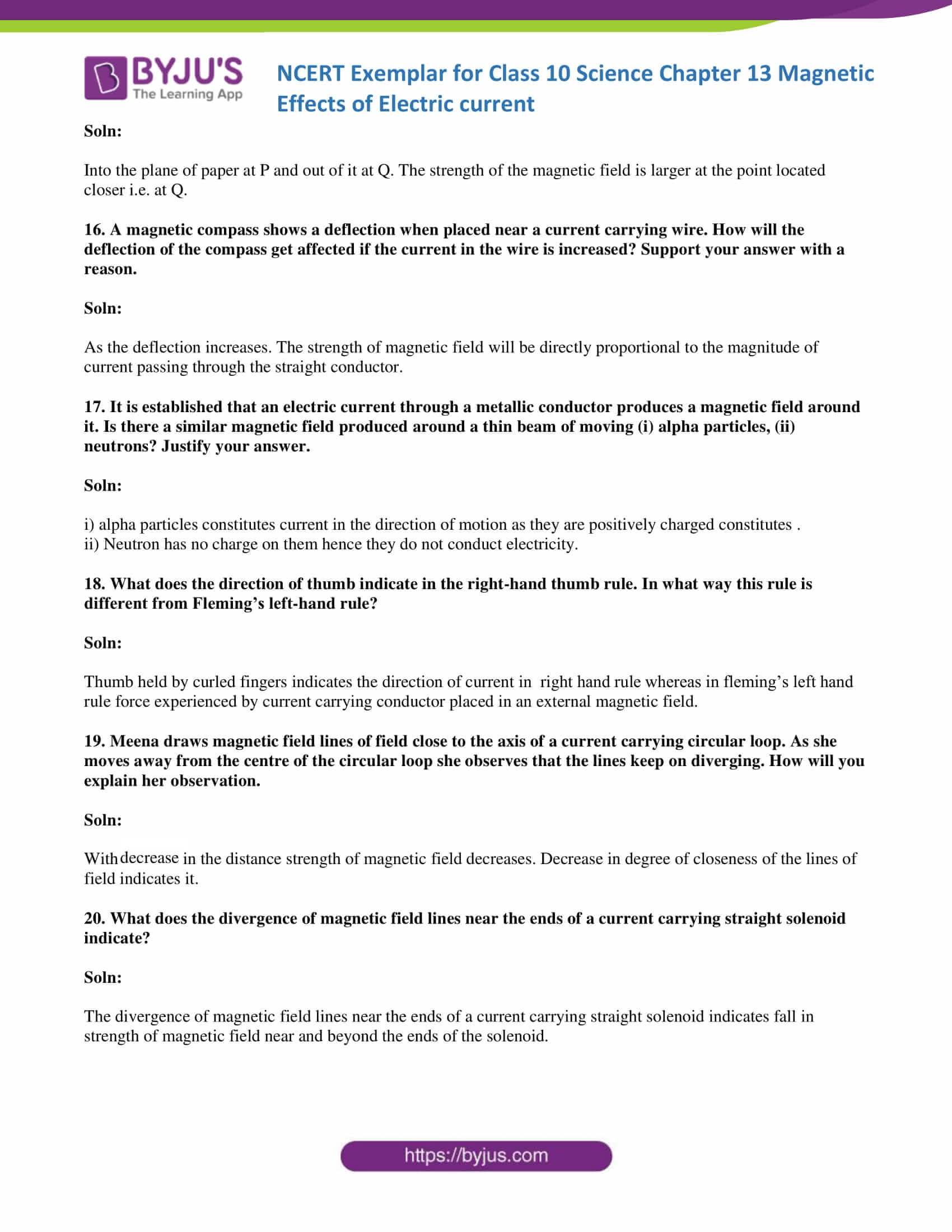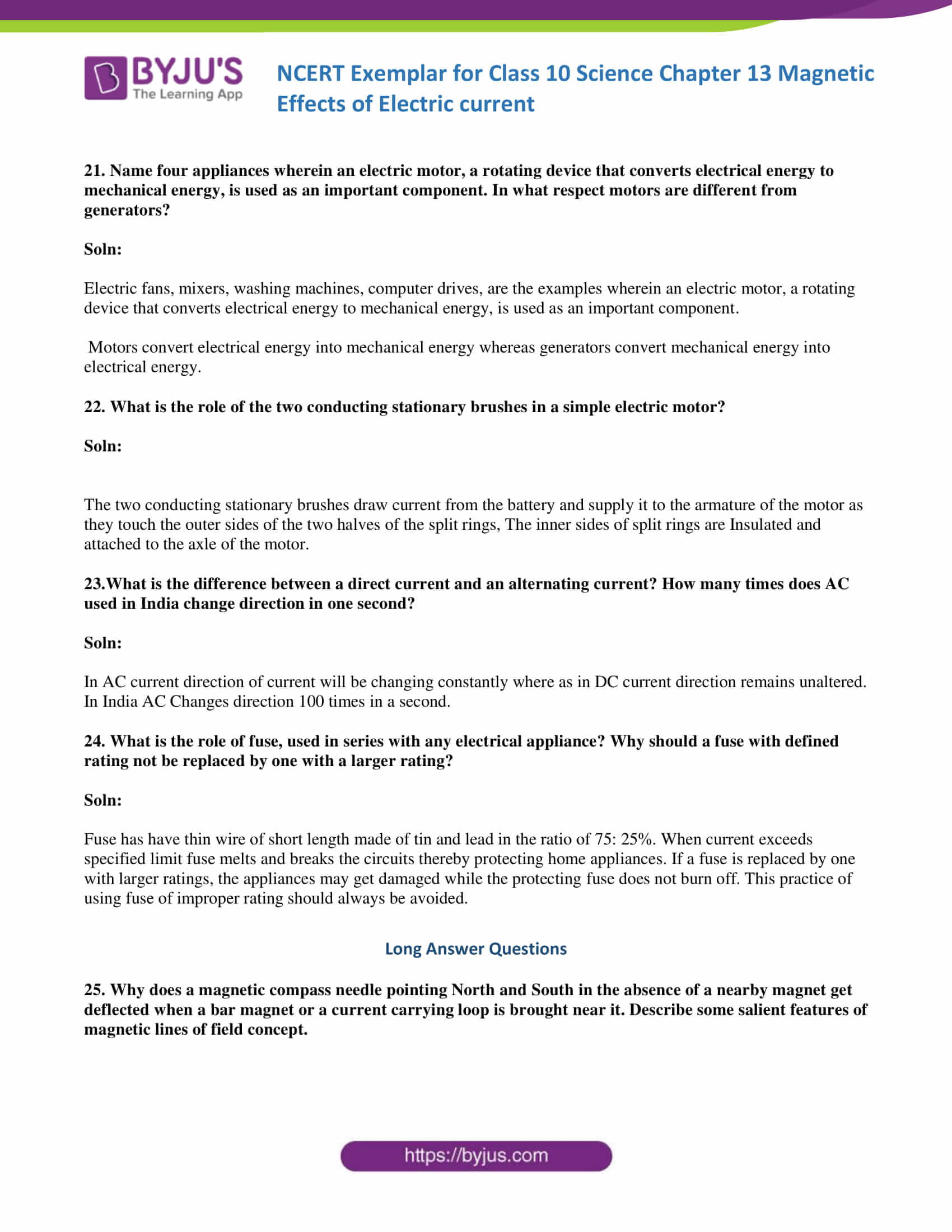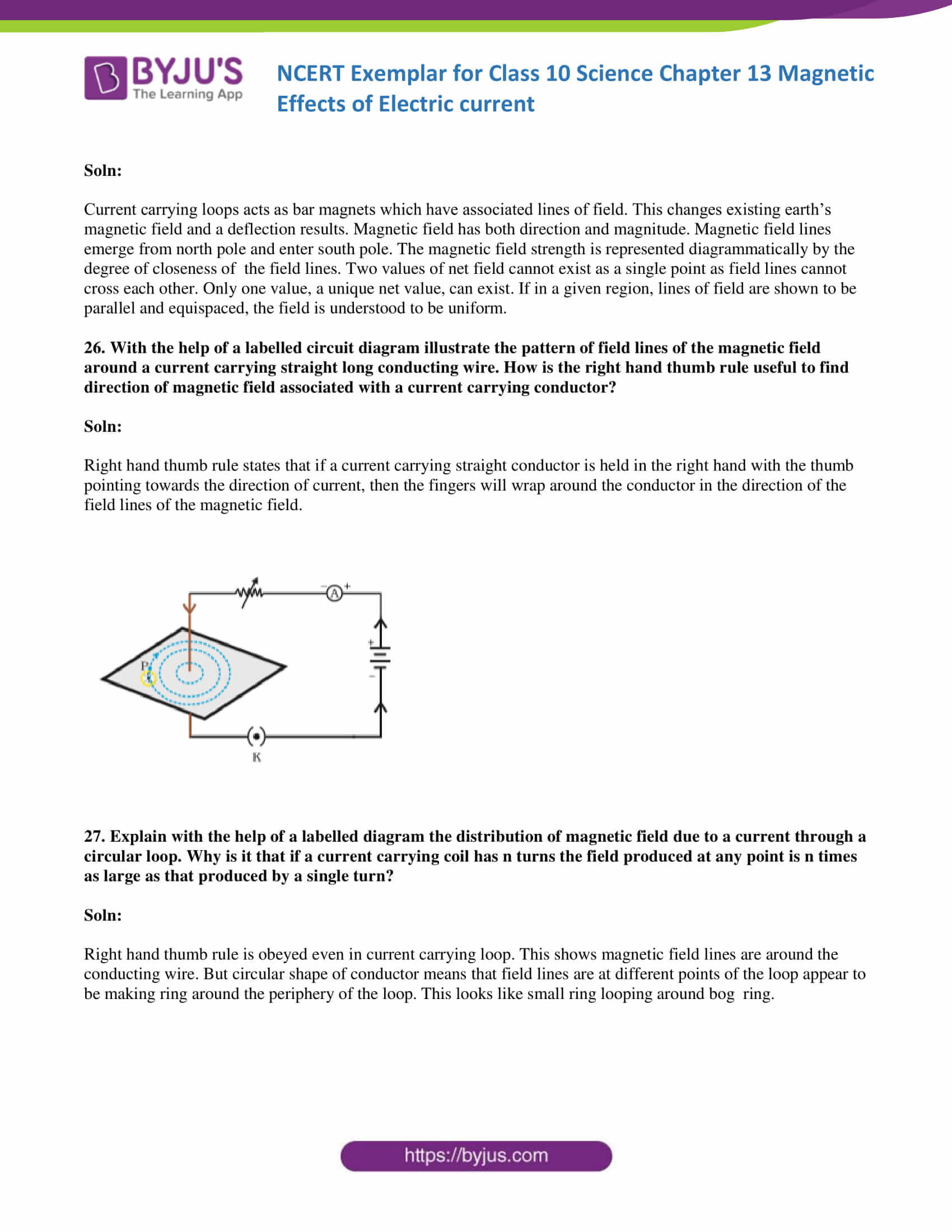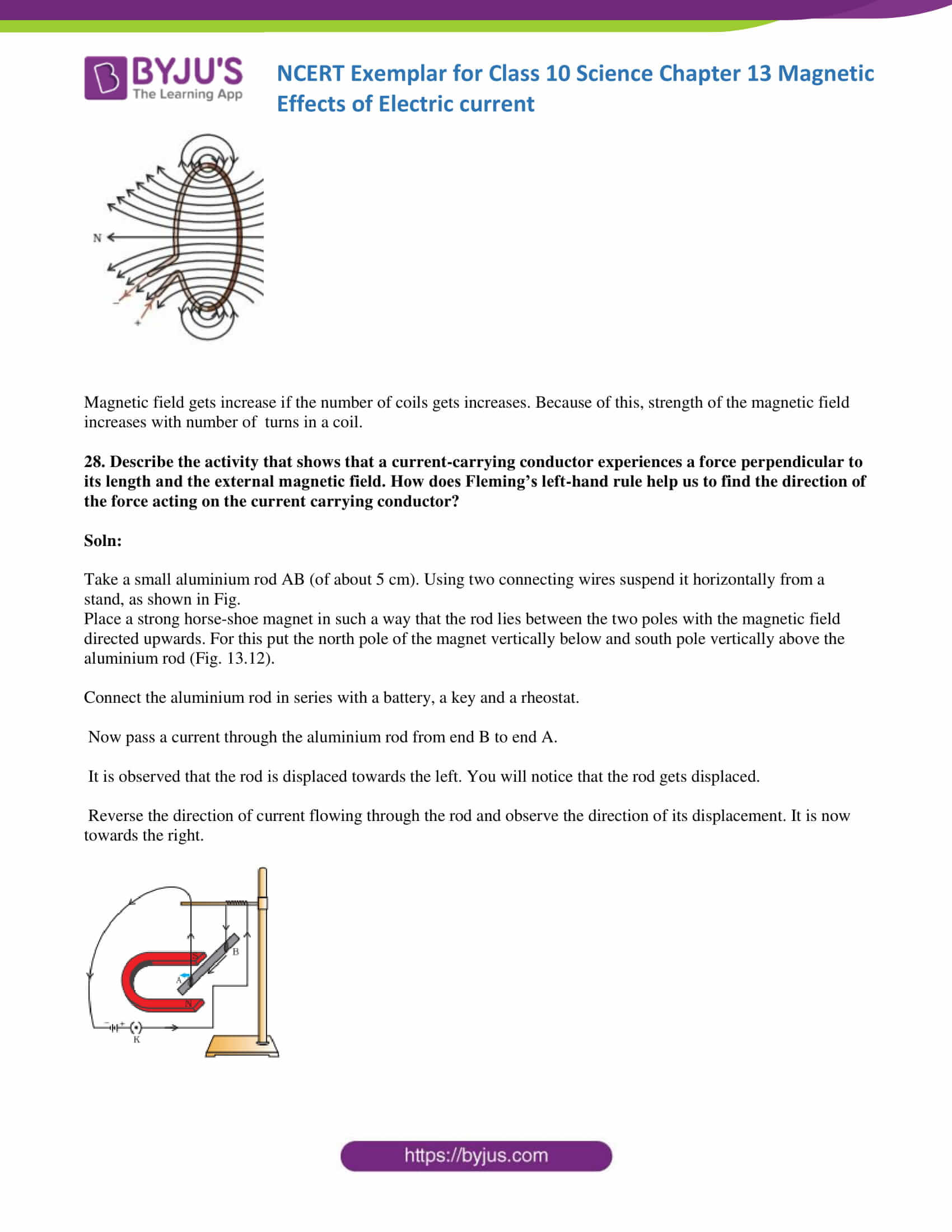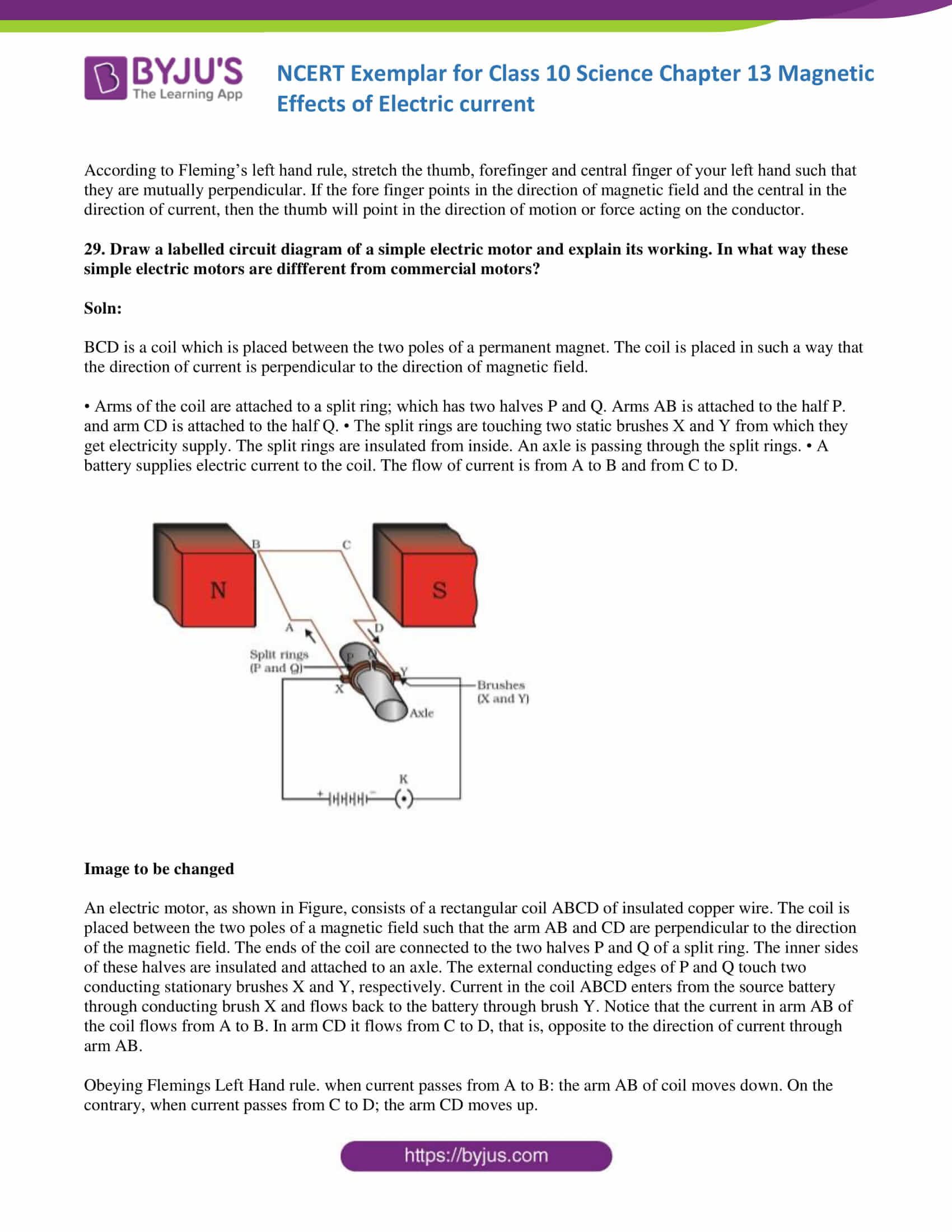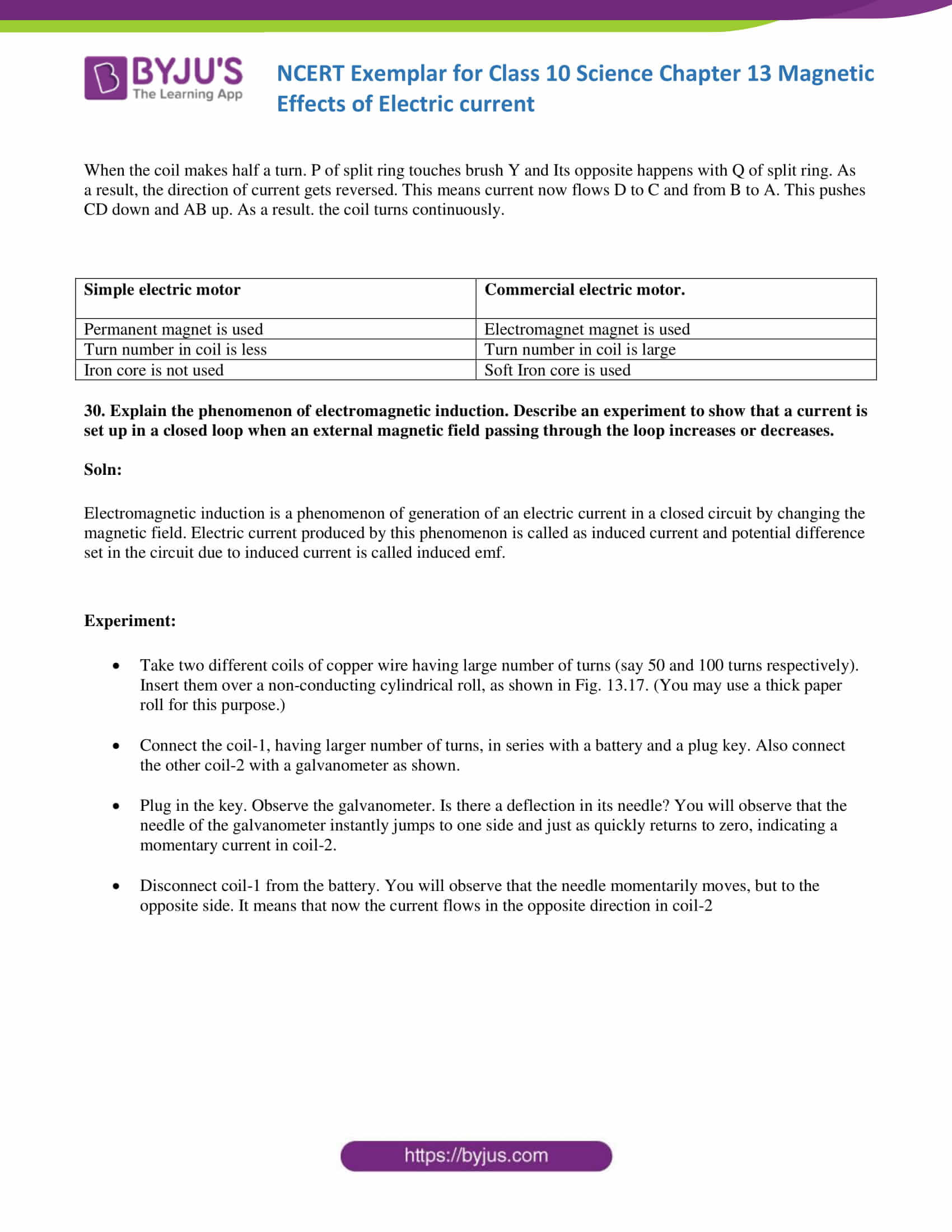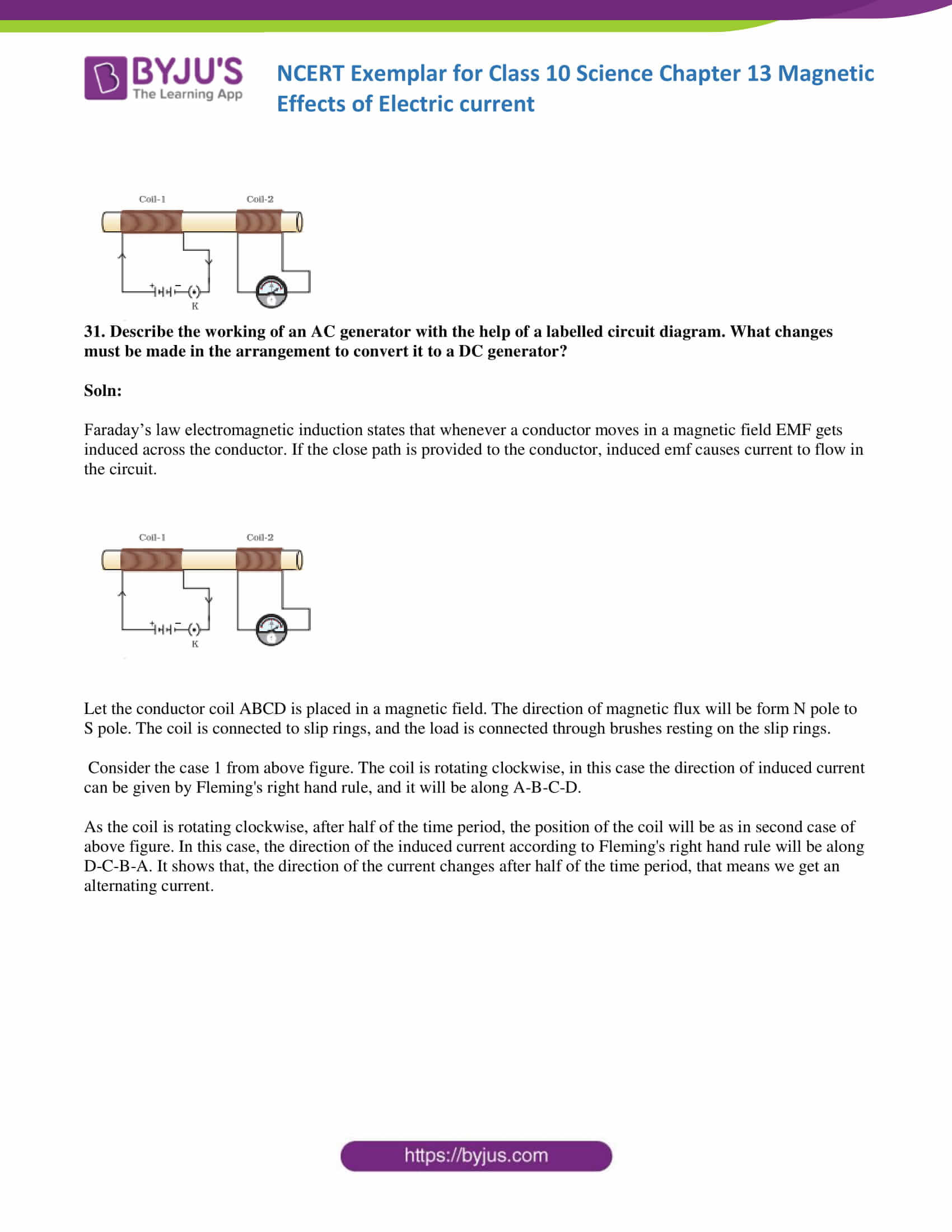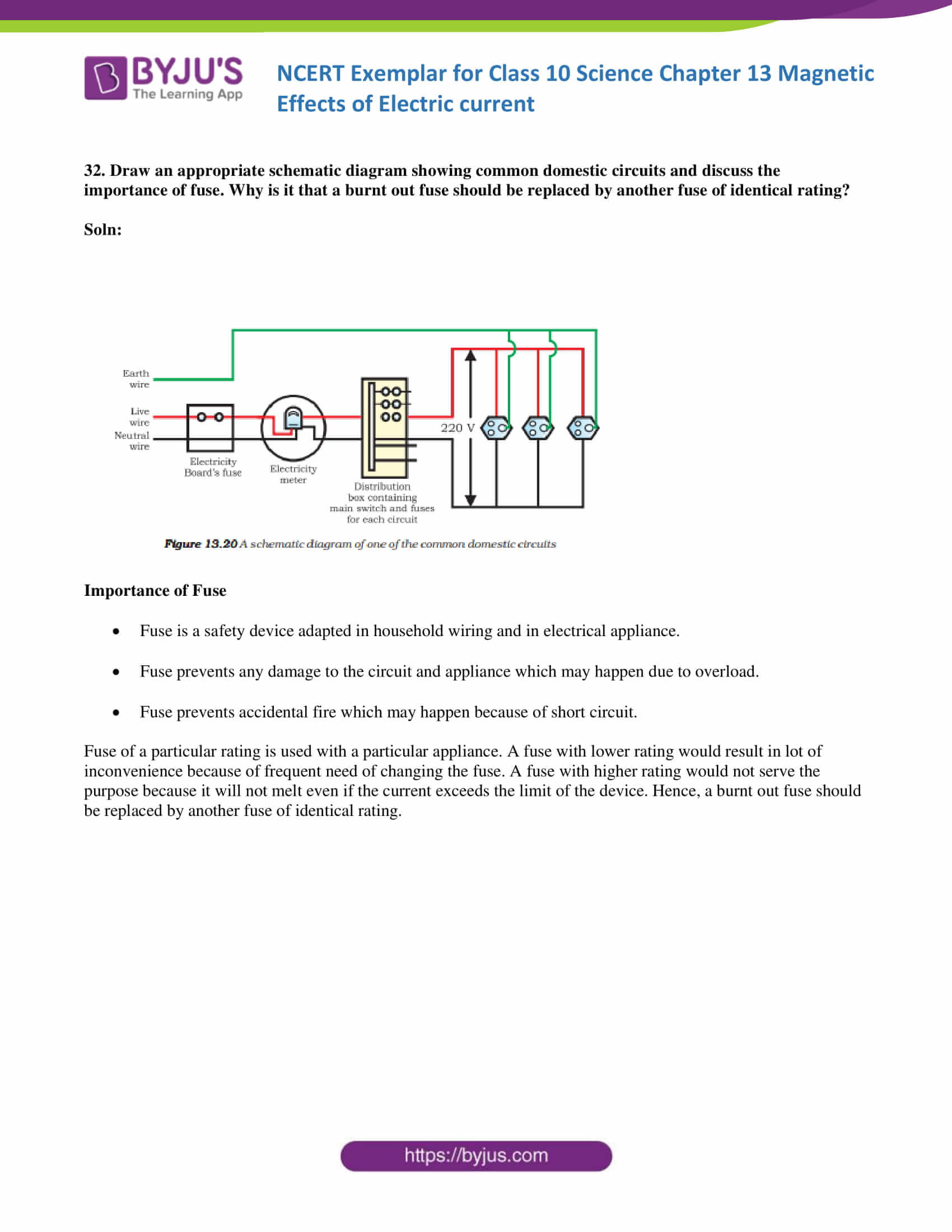### Multiple-choice Questions

1. Choose the incorrect statement from the following regarding magnetic lines of the field

(a) The direction of the magnetic field at a point is taken to be the direction in which the north pole of a magnetic compass needle points

(b) Magnetic field lines are closed curves

(c) If magnetic field lines are parallel and equidistant, they represent zero-field strength

(d) The relative strength of the magnetic field is shown by the degree of closeness of the field lines

Soln:

Answer is (c) If magnetic field lines are parallel and equidistant, they represent zero-field strength

Explanation:

Option c) is incorrect because parallel lines of magnetic field represent uniform magnetic field.

2. If the key in the arrangement (Figure 13.1) is taken out (the circuit is made open) and magnetic field lines are drawn over the horizontal plane ABCD, the lines are

(a) concentric circles

(b) elliptical in shape

(c) straight lines parallel to each other

(d) concentric circles near the point O but of elliptical shapes as we go away from it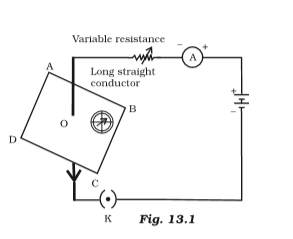Soln:

Explanation:

When open magnetic field lines are drawn over the horizontal plane ABCD, then the magnetic field lines will be in the form of concentric circles with centre at the axis of the conductor. This is because there will be no flow of current, and therefore, there will be no magnetic field due to the conductor. So, at point O, only Earth’s magnetic field will be present, so the magnetic field lines will be in the form of concentric circles.

3. A circular loop placed in a plane perpendicular to the plane of paper carries a current when the key is ON. The current, as seen from points A and B (in the plane of the paper and on the axis of the coil) is anti-clockwise and clockwise, respectively. The magnetic field lines point from B to A. The N-pole of the resultant magnet is on the face close to

(a) A

(b) B

(c) A if the current is small, and B if the current is large

(d) B if the current is small and A if the current is large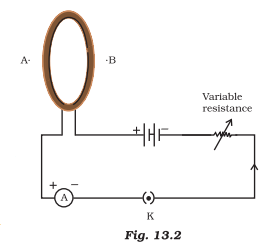Soln:

Explanation:

The direction of the magnetic field will be from south to north pole. Point A shows north pole because field lines are pointing from B to A.

4. For a current in a long straight solenoid N- and S-poles are created at the two ends. Among the following statements, the incorrect statement is

(a) The field lines inside the solenoid are in the form of straight lines, which indicates that the magnetic field is the same at all points inside the solenoid

(b) The strong magnetic field produced inside the solenoid can be used to magnetise a piece of magnetic material like soft iron, when placed inside the coil

(c) The pattern of the magnetic field associated with the solenoid is different from the pattern of the magnetic field around a bar magnet

(d) The N- and S-poles exchange position when the direction of current through the solenoid is reversed

Soln:

Answer is (c) The pattern of the magnetic field associated with the solenoid is different from the pattern of the magnetic field around a bar magnet

Explanation:

Solenoid behaves like Bar magnet, therefore, the pattern of the magnetic field associated with the solenoid is same as the pattern of the magnetic bar around a bar magnet.

5. A uniform magnetic field exists in the plane of paper pointing from left to right as shown in Figure 13.3. In the field an electron and a proton move as shown. The electron and the proton experience

(a) forces both pointing into the plane of paper

(b) forces both pointing out of the plane of paper

(c) forces pointing into the plane of paper and out of the plane of paper, respectively

(d) force pointing opposite and along the direction of the uniform magnetic field, respectively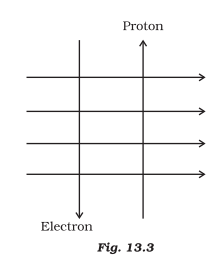Soln:

Answer is (a) forces both pointing into the plane of the paper

Explanation:

The direction of movement of electron is opposite to the direction of electric current. This will make the current move upwards. If the index finger shows the direction of magnetic field, ring finger shows the current direction and direction of thumb into paper.

6. Commercial electric motors do not use

(a) an electromagnet to rotate the armature

(b) effectively large number of turns of conducting wire in the current-carrying coil

(c) a permanent magnet to rotate the armature

(d) a soft iron core on which the coil is wound

Soln:

Answer is (c) a permanent magnet to rotate the armature

Explanation:

In electric motors Electromagnet is used instead of a permanent magnet.

7. In the arrangement shown in Figure 13.4, there are two coils wound on a non-conducting cylindrical rod. Initially, the key is not inserted. Then the key is inserted and later removed. Then

(a) the deflection in the galvanometer remains zero throughout

(b) there is a momentary deflection in the galvanometer but it dies out shortly and there is no effect when the key is removed

(c) there are momentary galvanometer deflections that die out shortly; the deflections are in the same direction

(d) there are momentary galvanometer deflections that die out shortly; the deflections are in opposite directions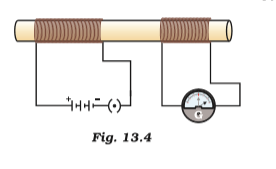Soln:

The answer is (d) there are momentary galvanometer deflections that die out shortly; the deflections are in opposite directions

Explanation:

When key is plugged galvanometer shows deflection in one direction and the direction of deflection reverses if we unplug the galvanometer.

8. Choose the incorrect statement

(a) Fleming’s right-hand rule is a simple rule to know the direction of induced current

(b) The right-hand thumb rule is used to find the direction of magnetic fields due to current-carrying conductors (c) The difference between the direct and alternating currents is that the direct current always flows in one direction, whereas the alternating current reverses its direction periodically

(d) In India, the AC changes direction after every 1/50 second

Soln:

The answer is (d) In India, the AC changes direction after every 1/50 second

Explanation:

AC frequency in India is 50 Hz. The direction changes twice in each cycle so that the change of direction takes place after every 1/00 second.

9. A constant current flows in a horizontal wire in the plane of the paper from east to west as shown in Figure 13.5. The direction of the magnetic field at a point will be North to South

(a) directly above the wire

(b) directly below the wire

(c) at a point located in the plane of the paper, on the north side of the wire

(d) at a point located in the plane of the paper, on the south side of the wire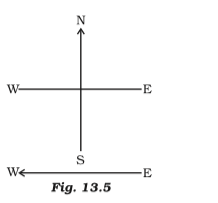Soln:

The answer is (b) directly below the wire

Explanation:

By applying right-hand thumb rule we can find that direction of the magnetic field is from North to South below the wire.

10. The strength of the magnetic field inside a long current carrying straight solenoid is

(a) more at the ends than at the centre

(b) minimum in the middle

(c) same at all points

(d) found to increase from one end to the other

Soln:

Answer is (c) same at all points

Explanation:

Inside the solenoid, magnetic field lines are straight. This indicates a strong magnetic field. Hence magnetic field is uniform at all points inside the solenoid.

11. To convert an AC generator into DC generator

(a) split-ring type commutator must be used

(b) slip rings and brushes must be used

(c) a stronger magnetic field has to be used

(d) a rectangular wire loop has to be used

Soln:

Answer is (a) split-ring type commutator must be used

Explanation:

Split-ring type commutator reverses the direction of current after each half-turn of the armature. This maintains a DC current.

12. The most important safety method used for protecting home appliances from short-circuiting or overloading is (a) earthing

(b) use of fuse

(c) use of stabilizers

(d) use of electric meter

Soln:

Answer is (b) use of fuse

Explanation:

Fuse has thin wire of short length made of tin and lead in the ratio of 75: 25%. When current exceeds specified limit fuse melts and breaks the circuits thereby protecting home appliances.

13. A magnetic compass needle is placed in the plane of paper near point A as shown in Figure 13.6. In which plane should a straight current-carrying conductor be placed so that it passes through A and there is no change in the deflection of the compass? Under what condition is the deflection maximum and why?

Soln:

In the plane of the paper itself. The axis of the compass is vertical and the field due to the conductor is also vertical. It could result in a dip of a compass needle which is not possible in this case (dips result only if the axis of the compass is horizontal). The deflection is maximum when the conductor through A is perpendicular to the plane of the paper and the field due to it is maximum in the plane of the paper.

14. Under what conditions permanent electromagnet is obtained if a current-carrying solenoid is used? Support your answer with the help of a labelled circuit diagram.

Soln:

To obtain a permanent electromagnet from a current-carrying solenoid following conditions are required.

1. Closed-circuit
2. Core of soft iron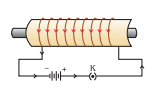15. AB is a current-carrying conductor in the plane of the paper as shown in Figure 13.7. What are the directions of magnetic fields produced by it at points P and Q? Given r1 > r2, where will the strength of the magnetic field be larger?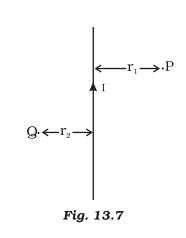Soln:

Into the plane of paper at P and out of it at Q. The strength of the magnetic field is larger at the point located closer i.e. at Q.

16. A magnetic compass shows a deflection when placed near a current-carrying wire. How will the deflection of the compass get affected if the current in the wire is increased? Support your answer with a reason.

Soln:

As the deflection increases. The strength of the magnetic field will be directly proportional to the magnitude of current passing through the straight conductor.

17. It is established that an electric current through a metallic conductor produces a magnetic field around it. Is there a similar magnetic field produced around a thin beam of moving (i) alpha particles, (ii) neutrons? Justify your answer.

Soln:

i) Alpha particles constitute the current in the direction of motion as they are positively charged constitutes.

ii) Neutron has no charge on them hence they do not conduct electricity.

18. What does the direction of thumb indicate in the right-hand thumb rule? In what way this rule is different from Fleming’s left-hand rule?

Soln:

The thumb held by curled fingers indicates the direction of current in right-hand rule, whereas in fleming’s left-hand rule, force is experienced by the current-carrying conductor placed in an external magnetic field.

19. Meena draws magnetic field lines of the field close to the axis of a current-carrying circular loop. As she moves away from the centre of the circular loop she observes that the lines keep on diverging. How will you explain her observation?

Soln:

With a decrease in the distance strength of the magnetic field decreases. The decrease in degree of closeness of the lines of field indicates it.

20. What does the divergence of magnetic field lines near the ends of a current-carrying straight solenoid indicate?

Soln:

The divergence of magnetic field lines near the ends of a current-carrying straight solenoid indicates fall in the strength of magnetic field near and beyond the ends of the solenoid.

21. Name four appliances wherein an electric motor, a rotating device that converts electrical energy to mechanical energy, is used as an important component. In what respect motors are different from generators?

Soln:

Electric fans, mixers, washing machines, and computer drives are examples wherein an electric motor, a rotating device that converts electrical energy to mechanical energy, is used as an important component.

Motors convert electrical energy into mechanical energy, whereas generators convert mechanical energy into electrical energy.

22. What is the role of the two conducting stationary brushes in a simple electric motor?

Soln:

The two conducting stationary brushes draw current from the battery and supply it to the armature of the motor as they touch the outer sides of the two halves of the split rings, The inner sides of split rings are Insulated and attached to the axle of the motor.

23. What is the difference between a direct current and an alternating current? How many times does AC used in India change direction in one second?

Soln:

In AC current direction of current will be changing constantly whereas in DC current direction remains unaltered. In India AC Changes direction 100 times in a second.

24. What is the role of fuse, used in series with any electrical appliance? Why should a fuse with defined rating not be replaced by one with a larger rating?

Soln:

Fuse has have thin wire of short length made of tin and lead in the ratio of 75: 25%. When current exceeds specified limit fuse melts and breaks the circuits thereby protecting home appliances. If a fuse is replaced by one with larger ratings, the appliances may get damaged while the protecting fuse does not burn off. This practice of using a fuse of improper rating should always be avoided.

25. Why does a magnetic compass needle pointing North and South in the absence of a nearby magnet get deflected when a bar magnet or a current-carrying loop is brought near it. Describe some salient features of magnetic lines of field concept.

Soln:

Current carrying loops act as bar magnets which have associated lines of the field. This changes the existing earth’s magnetic field and a deflection results. Magnetic field has both direction and magnitude. Magnetic field lines emerge from the north pole and enter the south pole. The magnetic field strength is represented diagrammatically by the degree of closeness of the field lines. Two values of net field cannot exist as a single point as field lines cannot cross each other. Only one value, a unique net value, can exist. If in a given region, lines of field are shown to be parallel and equispaced, the field is understood to be uniform.

26. With the help of a labelled circuit diagram illustrating the pattern of field lines of the magnetic field around a current-carrying straight long conducting wire. How is the right-hand thumb rule useful to find the direction of the magnetic field associated with a current-carrying conductor?

Soln:

Right-hand thumb rule states that if a current-carrying straight conductor is held in the right hand with the thumb pointing towards the direction of current, then the fingers will wrap around the conductor in the direction of the field lines of the magnetic field.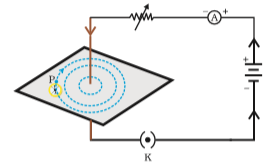27. Explain with the help of a labelled diagram the distribution of magnetic field due to a current through a circular loop. Why is it that if a current-carrying coil has n turns the field produced at any point is n times as large as that produced by a single turn?

Soln:

Right hand thumb rule is obeyed even in current-carrying loop. This shows magnetic field lines are around the conducting wire. But circular shape of conductor means that field lines at different points of the loop appear to be making a ring around the periphery of the loop. This looks like small ring looping around the periphery of big ring.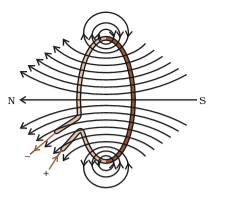Magnetic field gets increase if the number of coils gets increases. Because of this, the strength of the magnetic field increases with the number of turns in a coil.

28. Describe the activity that shows that a current-carrying conductor experiences a force perpendicular to its length and the external magnetic field. How does Fleming’s left-hand rule help us to find the direction of the force acting on the current-carrying conductor?

Soln:

Take a small aluminium rod AB (of about 5 cm). Using two connecting wires suspend it horizontally from a stand, as shown in Fig.

Place a strong horseshoe magnet in such a way that the rod lies between the two poles with the magnetic field directed upwards. For this put the north pole of the magnet vertically below and south pole vertically above the aluminium rod (Fig. 13.12).

Connect the aluminium rod in series with a battery, a key and a rheostat.

Now pass a current through the aluminium rod from end B to end A.

It is observed that the rod is displaced towards the left. You will notice that the rod gets displaced.

Reverse the direction of current flowing through the rod and observe the direction of its displacement. It is now towards the right.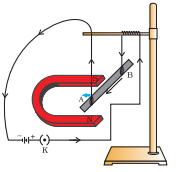According to Fleming’s left-hand rule, stretch the thumb, forefinger and central finger of your left hand such that they are mutually perpendicular. If the forefinger points in the direction of the magnetic field and the central in the direction of current, then the thumb will point in the direction of motion or force acting on the conductor.

29. Draw a labelled circuit diagram of a simple electric motor and explain its working. In what way these simple electric motors are different from commercial motors?

Soln:

BCD is a coil which is placed between the two poles of a permanent magnet. The coil is placed in such a way that the direction of the current is perpendicular to the direction of the magnetic field.

• Arms of the coil are attached to a split ring; which has two halves P and Q. Arms AB is attached to the half P. and arm CD is attached to the half Q. • The split rings are touching two static brushes X and Y from which they get electricity supply. The split rings are insulated from inside. An axle is passing through the split rings. • A battery supplies electric current to the coil. The flow of current is from A to B and from C to D.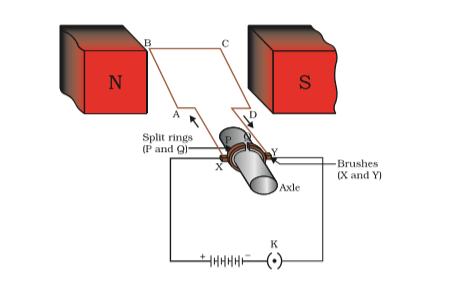Image to be changed

An electric motor, as shown in Figure, consists of a rectangular coil ABCD of insulated copper wire. The coil is placed between the two poles of a magnetic field such that the arm AB and CD are perpendicular to the direction of the magnetic field. The ends of the coil are connected to the two halves P and Q of a split ring. The inner sides of these halves are insulated and attached to an axle. The external conducting edges of P and Q touch two conducting stationary brushes X and Y, respectively. Current in the coil ABCD enters from the source battery through conducting brush X and flows back to the battery through brush Y. Notice that the current in arm AB of the coil flows from A to B. In arm CD it flows from C to D, that is, opposite to the direction of current through arm AB.

Obeying Fleming’s Left-Hand rule. when current passes from A to B: the arm AB of the coil moves down. On the contrary, when current passes from C to D; the arm CD moves up.

When the coil makes half a turn. P of split ring touches brush Y and Its opposite happens with Q of split ring. As a result, the direction of current gets reversed. This means current now flows D to C and from B to A. This pushes CD down and AB up. As a result. the coil turns continuously.

 Simple electric motor Commercial electric motor. Permanent magnet is used Electromagnet magnet is used Turn number in coil is less Turn number in coil is large Iron core is not used Soft Iron core is used

30. Explain the phenomenon of electromagnetic induction. Describe an experiment to show that a current is set up in a closed loop when an external magnetic field passing through the loop increases or decreases.

Soln:

Electromagnetic induction is a phenomenon of generation of an electric current in a closed circuit by changing the magnetic field. Electric current produced by this phenomenon is called as induced current and potential difference set in the circuit due to induced current is called induced emf.

Experiment:

• Take two different coils of copper wire having a large number of turns (say 50 and 100 turns respectively). Insert them over a non-conducting cylindrical roll, as shown in Fig. 13.17. (You may use a thick paper roll for this purpose.)
• Connect the coil-1, having a larger number of turns, in series with a battery and a plug key. Also, connect the other coil-2 with a galvanometer as shown.
• Plugin the key. Observe the galvanometer. Is there a deflection in its needle? You will observe that the needle of the galvanometer instantly jumps to one side and just as quickly returns to zero, indicating a momentary current in coil-2.
• Disconnect coil-1 from the battery. You will observe that the needle momentarily moves, but to the opposite side. It means that now the current flows in the opposite direction in coil-2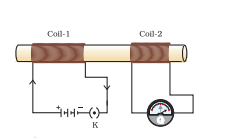31. Describe the working of an AC generator with the help of a labelled circuit diagram. What changes must be made in the arrangement to convert it to a DC generator?

Soln:

Faraday’s law of electromagnetic induction states that whenever a conductor moves in a magnetic field EMF gets induced across the conductor. If the close path is provided to the conductor, induced emf causes current to flow in the circuit.Let the conductor coil ABCD is placed in a magnetic field. The direction of magnetic flux will be form N pole to S pole. The coil is connected to slip rings, and the load is connected through brushes resting on the slip rings.

Consider case 1 from the above figure. The coil is rotating clockwise, in this case, the direction of induced current can be given by Fleming’s right-hand rule, and it will be along A-B-C-D.

As the coil is rotating clockwise, after half of the time period, the position of the coil will be as in the second case of the above figure. In this case, the direction of the induced current, according to Fleming’s right-hand rule will be along D-C-B-A. It shows that the direction of the current changes after half of the time period, which means we get an alternating current.

32. Draw an appropriate schematic diagram showing common domestic circuits and discuss the importance of fuse. Why is it that a burnt-out fuse should be replaced by another fuse of identical rating?

Soln: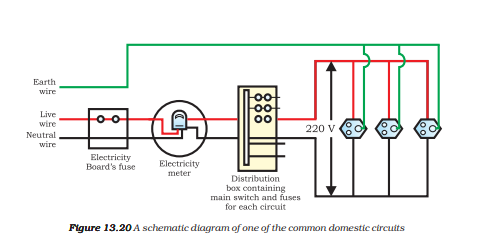Importance of Fuse

• Fuse is a safety device adapted to household wiring and electrical appliance.
• Fuse prevents any damage to the circuit and appliance which may happen due to overload.
• Fuse prevents accidental fire, which may happen because of a short circuit.

The Fuse of a particular rating is used with a particular appliance. A fuse with a lower rating would result in a lot of inconveniences because of the frequent need to change the fuse. A fuse with a higher rating would not serve the purpose because it will not melt even if the current exceeds the limit of the device. Hence, a burnt-out fuse should be replaced by another fuse of identical rating.

### Class 10 Science Chapter 13 Magnetic Effects of Electric Current

To facilitate seamless learning and help students understand the chapter more clearly, the free NCERT Exemplar Solutions for Class 10 Science Chapter 13 are provided here in the form of a downloadable PDF. Students can use these solutions as an effective reference tool to study productively and be ready with proper answers for any question that can be asked in the board examination.

Important Concepts Covered in NCERT Exemplar for Class 10 Magnetic Effects of Electric Current

• Magnetic field and field lines
• Magnetic field due to current carrying conductor
• Magnetic field due to the current through a straight conductor
• Right-hand thumb rule
• Magnetic field due to a current through a circular loop
• Magnetic field due to a current in a solenoid
• Force on a current-carrying conductor in a magnetic field
• Electric motor
• Electromagnetic induction
• Electric generator
• Domestic electric circuits

Class 10 Science Chapter 13, Magnetic Effects of Electric Current, has been allotted high marks in the CBSE Class 10 board examination. In order to excel in exams, students are advised to solve this NCERT Exemplar. To get the best study materials, notes, previous years’ questions, sample papers and chapter-wise NCERT exemplars, visit BYJU’S website or Download BYJU’S – The Learning App.

## Frequently Asked Questions on NCERT Exemplar Solutions for Class 10 Science Chapter 13

Q1

### What are the topics and subtopics present in Chapter 13 of NCERT Exemplar Solutions for Class 10 Science?

The topics and subtopics present in Chapter 13 of NCERT Exemplar Solutions for Class 10 Science are listed below:
Magnetic field and field lines
Magnetic field due to current carrying conductor
Magnetic field due to the current through a straight conductor
Right-hand thumb rule
Magnetic field due to a current through a circular loop
Magnetic field due to a current in a solenoid
Force on a current-carrying conductor in a magnetic field
Electric motor
Electromagnetic induction
Electric generator
Domestic electric circuits
Q2

### How can I score full marks in Chapter 13 of NCERT Exemplar Solutions for Class 10 Science?

The NCERT Exemplar Solutions for Class 10 Science Chapter 13 are prepared by highly experienced faculty after conducting vast research on each concept. While solving the textbook questions, students can refer to these solutions to clear their doubts  as well as to get an overall idea about the topics covered in this chapter. The solutions can be accessed in both online and offline modes as per the needs of students. The PDF of these solutions is available on BYJU’S website, which can be downloaded and used by the students to get their doubts cleared instantly.
Q3

### Explain the importance of fuse covered in Chapter 13 of NCERT Exemplar Solutions for Class 10 Science.

Importance of Fuse
1. Fuse is a safety device used in household wiring and in electrical appliances.
2. Fuse prevents any damage to the circuit and appliance, which may happen due to overload.
3. It prevents accidental fire, which may happen because of a short circuit.
Students of Class 10 are advised to refer to the NCERT Solutions from BYJU’S to gain a grip on the important concepts. The solutions are prepared with the main aim of helping students with their exam preparation. The stepwise explanations in simple language boost the confidence of students to attempt the board exam without fear.